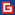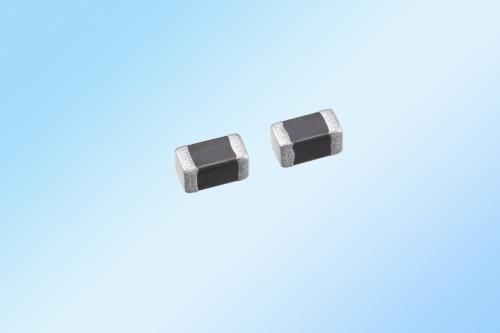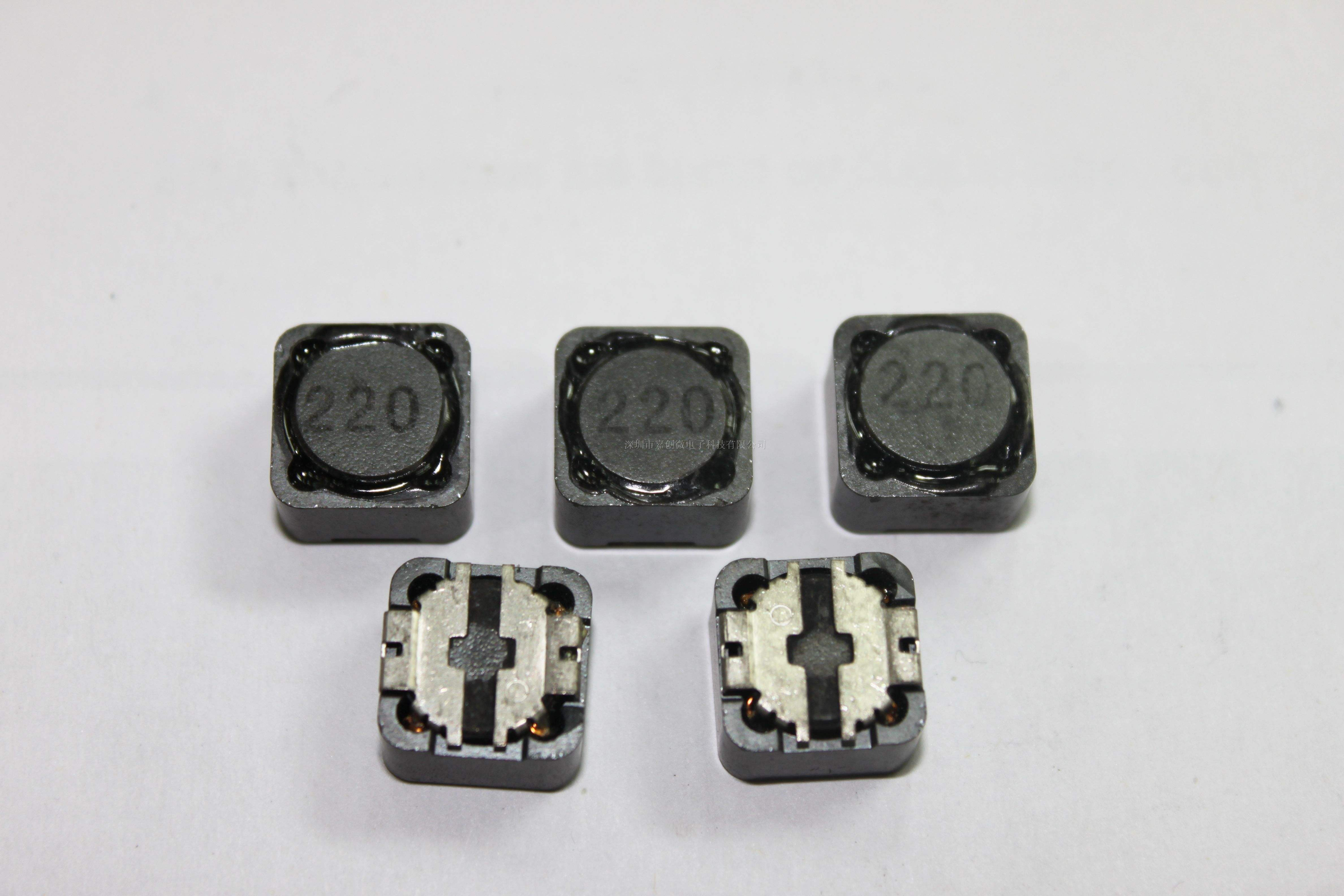# Inventory: How much electricity does the switch light consume instantaneously?

When I was a child, I heard the adults say, "Donâ€™t always turn on the lights and turn off the lights. Itâ€™s the most expensive to turn on the lights." At that time, the house used an incandescent light bulb. When I learned the physics and understood the voltage and current resistance, I realized that the incandescent lamp converts the electric energy into heat energy. Although the temperature of the filament is low at the moment of turning on the light, the resistance is only about one tenth of the normal working. Therefore, it consumes more power. However, this process is only a few seconds, but it consumes more than one minute of stable light, and there is no need to pay much.

Now, everyone is lighting energy-saving lamps such as fluorescent lamps, which are more energy efficient and more energy efficient. Some lights require a very high voltage to warm up when starting, and the voltage of the external circuit is constant. This high-voltage start is usually achieved by the internal circuit of the lamp, such as the starter of the fluorescent tube.

Perhaps because of the preheating, the saying on the rivers and lakes that the "turning the lights on and off the most electricity" was put on the energy-saving lamps, saying that "the switch is equivalent to continuously lighting for N hours." Some time ago, an article about "Earth Hour" in "New Knowledge Weekly" mentioned this rumor, where N is equal to 1. And in some online posts, this N is even equal to 10!

At first glance, this argument seems quite reasonable. However, if it is really equivalent to 10 hours of power consumption, in the case of a 20-watt bulb, the instant power consumption will be as high as 720 kilojoules, enough to boil 2 liters of water. This large amount of heat is dissipated in a very short period of time. When we turn on the lights, we donâ€™t feel the heat waves coming from the surface. Isnâ€™t it too strange?

So, how much power does the energy-saving light switch consume in the end? We use an experiment to answer this question. We built a power-saving bulb (EDXO-14 integrated fluorescent lamp) with a rated voltage of 120 volts (60 Hz, US), a power rating of 14 watts, and some original circuits (resistors, multimeters, and oscilloscopes). a circuit. Among them, a small resistor (13.6 ohms) and energy-saving bulbs are connected in series to a 120 volt, 60 Hz AC power source. By measuring the voltage across the resistor, we can calculate the current through the energy-saving bulb to calculate the power of the bulb.

First, it is easy to measure the power of a light-saving bulb during stable operation. When the energy-saving lamp is normally illuminated after the switch is closed, the voltage across the resistor can be measured as 1.4 volts by using an ordinary multimeter. At the same time, we measured the external circuit voltage as 119.8 volts. Therefore the current through the bulb is 0.103 amps. Then the power of this bulb in stable operation is P = UI = (119.8 - 1.4) Ã— 0.103 â‰ˆ 12.2 watts, slightly lower than the 14 watts identified on the bulb.

However, the "high voltage" startup problem that we are concerned about is not fully displayed on ordinary universal meters. We tried to open the closed switch several times and there was no abnormality in the reading of the multimeter. This shows that the initial high voltage start-up time is very short, much less than the response time of the multimeter. Therefore, we have to use another instrument that can see short-term signals: an oscilloscope.

At the moment of turn-on, we saw the long-awaited â€œhigh-voltage start-upâ€â€”a very short pulse peak appeared on the oscilloscope's waveform. This represents the â€œhigh pressure startâ€ process of energy saving lamps. So how much power is consumed in this process?

In fact, this spike occurred within 1 millisecond (0.001 seconds) and the waveform was normal. This instantaneous high current is about 5 to 7 times that of normal operation. At 7 times, we can estimate that the energy-saving bulb consumes no more than 0.1 joules of electricity during this time, which is equivalent to less than 0.01 seconds of energy consumption under stable operation. Due to experimental limitations, the actual energy consumption may be somewhat different from the estimated value, but even a 10x amplification is equivalent to 0.1 second of energy consumption - the energy consumed to start is negligible.

From this experiment, we can see that the rumored energy-saving light switch instantly charges a lot of electricity is completely untenable. The total amount of energy consumed by the energy-saving lamp during startup is actually very small, and there is no case that the switch is continuously illuminated for several hours. You don't have to turn off the lights to save power.

Of course, switching the appliance too frequently will definitely affect the life of the appliance. From this point of view, it is reasonable to avoid switching the energy-saving lamps too frequently.An inductor is characterized by its inductance, which is the ratio of the voltage to the rate of change of current. In the International System of Units (SI), the unit of inductance is the henry (H) named for 19th century American scientist Joseph Henry. SMD Ferrite Core Power Inductor,SMD Multilayer Ceramic Shielding,Inductor Ferrite Bead Indcutor,Wire Wound SMD Power Inductor .In the measurement of magnetic circuits, it is equivalent to weber/ampere. Inductors have values that typically range from 1 ÂµH (10âˆ’6 H) to 20 H. Many inductors have a magnetic core made of iron or ferrite inside the coil, which serves to increase the magnetic field and thus the inductance.SMD Chip Inductor

SMD Ferrite Core Power Inductor, SMD Multilayer Ceramic Shielding, Inductor Ferrite Bead Indcutor, Wire Wound SMD Power Inductor

JINAN EMI SHIELDING TECHNOLOGY CO., LTD. , http://www.emirfi.com